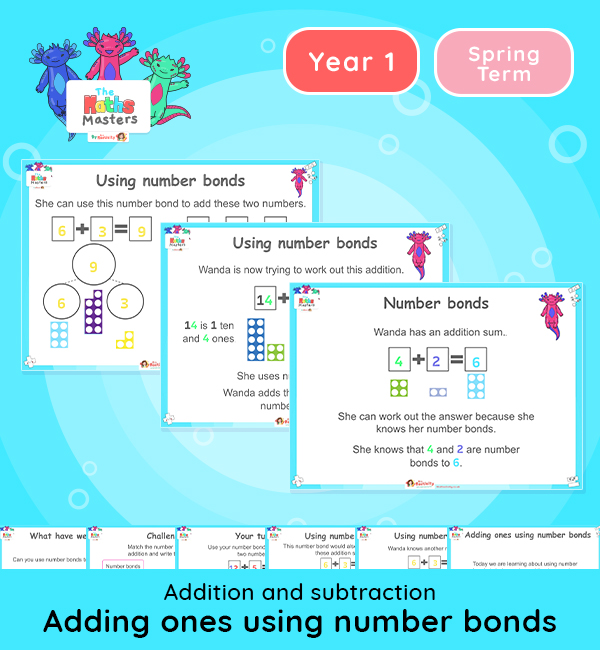# Year 1 | Adding Ones Using Number Bonds Lesson Presentation## White Rose Year 1 addition and subtraction lesson plans

Aligned with the White Rose Maths scheme of work, this Year 1 |Adding Ones Using Number Bonds Lesson Presentation is fully editable, and is designed for the Year 1 maths curriculum covering the following maths objectives for the autumn term:

This lesson practises using number bonds to add ones for numbers up to 20.

You may wish to spread this lesson over two sessions. Spend one day recapping numbers bonds within 10 and finding related additions to 20 and another working out the answers to additions within 20 using number bonds.

White Rose Maths Small Steps: Add ones using number bonds.

NC Links: Represent and use number bonds and related subtraction facts within 20. * Read, write and interpret mathematical statements involving addition, subtraction and equals signs. *Add and subtract one-digit and two-digit numbers to 20, including zero.

TAF Statements: Working towards: Add and subtract (one digit numbers) explaining their method verbally, in pictures, or using apparatus.

Working At: Recall all the number bonds to and within 10 and use these to reason with and calculate bonds to and within 20, recognising other associated additive relationships.

Greater Depth – Use reasoning about number and relationships to solve more complex problems and explain their thinking.

Ready-to-progress criteria:  1NF-1 Develop fluency in addition and subtraction facts within 10

Future applications: Add and subtract across 10.

1AS-2 Read, write and interpret equations containing addition(+), subtraction (-), and equals (=) symbols, and relate additive expressions and equations to real-life contexts.

## Recently Viewed